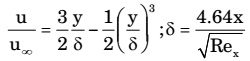## Fluid Mechanics and Hydraulic Machinery Miscellaneous

#### Fluid Mechanics and Hydraulic Machinery

1. An incompressible fluid flows over a flat plate with zero pressure gradient. The boundary layer thickness is 1 mm at a location where the Reynolds number is 1000. If the velocity of the fluid alone is increased by a factor of 4, then the boundary layer thickness at the same location, in mm will be

1.  Boundary layer thickness, δ ∝ 1 √Re

 Re ∝ V ⇒ δ ∝ 1 √V

##### Correct Option: C

 Boundary layer thickness, δ ∝ 1 √Re

 Re ∝ V ⇒ δ ∝ 1 √V

1. Consider an incompressible laminar boundary layer flow over a flat plate of length L, aligned with the direction of an incoming uniform free stream. If F is the ratio of the drag force on the front half of the plate to the drag force on the rear half, then

1. NA

##### Correct Option: D

NA

1. For air flow over a flat plate, velocity (U) and boundary layer thickness (δ) can be expressed respectively, asIf the free stream velocity is 2 m/s, and air has kinematic viscosity of 1.5 × 10-5 m2/s and density of 1.23 kg/m3, the wall shear stress at x = 1 m, is

1. NA

##### Correct Option: C

NA

1. If x is the distance measured from the leading edge of a flat plate, then laminar boundary layer thickness varies as

1.  ρ = 5x for laminar flow √Re

 Rex = Vx υ

 ∴ ρ = 5√x for laminar flow √V / υ

∴ ρ ∝ √x

##### Correct Option: D

 ρ = 5x for laminar flow √Re

 Rex = Vx υ

 ∴ ρ = 5√x for laminar flow √V / υ

∴ ρ ∝ √x

1. Flow separations flow past a solid object is caused by

1. NA

NA